|

# 坐站交替式健康办公家具选购全攻略

很多人都知道久坐不动是健康的大敌，但是危害究竟有多大并不清楚，以下列举几个数据便于理解。

有人疑问，现在的办公和娱乐基本都使用电脑、电视和手机，尤其是电脑更是不得不坐，怎么办呢？全球正在流行站式办公或者被称之为坐站交替式办公，使用可升降的办公家具达到坐累了站起来工作、站累了坐下来工作的目的，值得国内倍受久坐荼毒的人士借鉴。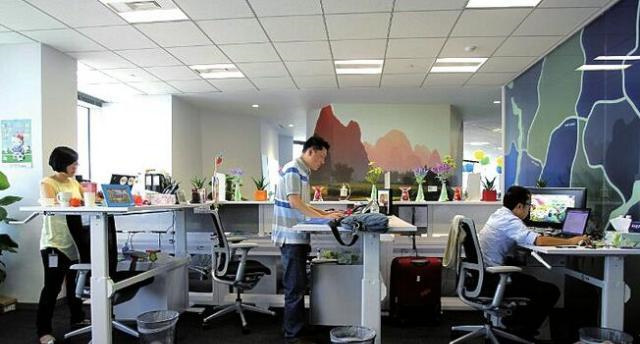为什么站式办公这么流行？仅举一个简单的调查数据，人站着比坐着消耗的热量多3－5倍，站立一小时相当于跑步一公里。假设一天工作8小时，其中4小时用站姿工作，那么相当于一天跑步4公里，而跑步4公里对身体的健康起到的作用不言而喻。站式办公家具有很多种类，究竟该如何挑选呢？

一、国际主流站立式办公家具的四种样式

谈起站式办公家具不得不提美国的爱格升公司，英文名Ergotron，这家公司由Harry Sweere于1982年在美国明尼苏达州建立，34年时间里，爱格升研制出种类繁多的人机界面交互产品，设计独特，功能实用，覆盖办公室、医疗机构、教育、制造/销售、家庭等各种应用场景，是中国许多显示器支架品牌的共同老师。

Ergotron进入中国市场遇到了“强龙难压地头蛇”的尴尬，国产质次价低的仿品抢走了不少低端消费者，但是仍无法撼动爱格升高端市场的地位。为了进一步开拓国内市场，为中国客户提供更加优质完善的产品，爱格升在中国实行了代理商制度，2017年达宝利与戴尔、联想一起续约成为爱格升的金牌代理商。

在站立式办公家具方面，爱格升早于追随者们设计了多款形态的产品，其中最为经典的有四款。第三图Ergotron WorkFit-A支臂式坐站两用工作站。它的优点是与桌面仅有一个支臂接触点，几乎不占桌面空间，显示器和键盘、鼠标均可悬空操作，不仅能垂直升降，而且可以左右移动，适合经常需要电脑展示的场景。

第四图Ergotron Workfit-S，工作原理是将一个升降支架牢固的固定在桌面上，支架附带显示器支臂和键鼠板，只需用很小的力升降工作台即可切换坐站姿势。键鼠板与显示器之间的高度可调、

第五图Ergotron WorkFit-TL坐站两用桌面工作台。它的优点是无需组装，只需放置在桌面上，将显示器和键鼠摆放其上，即可便捷的实现坐站交替办公。不用时，只需将它折叠收起便可腾空桌面，由于体积小，成本和价格也更低一些。

第七图Ergotron Workfit-D坐站两用自助工作台。它是一张采用CF恒力技术制造的升降桌，只需轻轻一碰按钮就可迅速调节桌面的高度，瞬间切换坐姿和站姿。它的优点是桌面面积大，承重力强，适合工作资料种类繁多的场景。

经过长期的市场检测，放置于原有桌面上使用的坐站两用桌面工作台（例WorkFit-TL）和升降桌类产品（例Workfit-D）成为市场站式办公家具的主流型产品。

二、坐站升降办公桌的分类和特点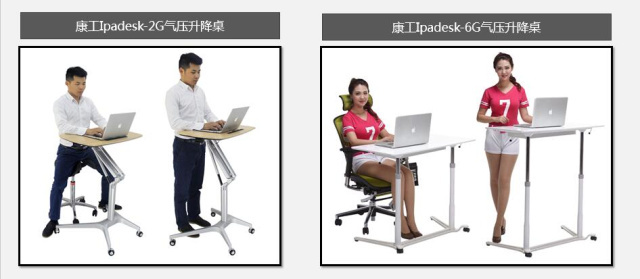前者精致小巧，适宜使用笔记本电脑、书写、当作演讲桌或放置投影移等静止物品使用。后者适宜台式电脑等较重的办公设备使用。前者在机动性方面强于后者，而后者在稳定性和承重力方面胜于前者。

升降办公桌从升降方式划分，可分为气棒式、手摇式和电动式三种。例如爱格升Workfit-D气棒式坐站升降桌、达宝利手摇式坐站升降桌和达宝利智能电动式坐站升降桌。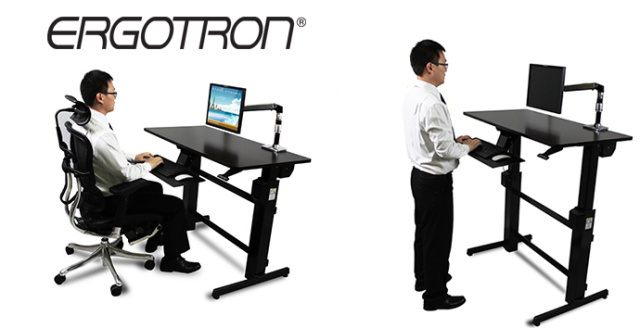气棒式升降桌的优点是调节方便，升降速度快。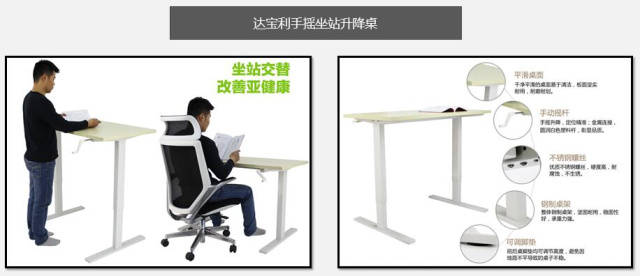手摇式升降桌的优点是纯机械结构，安全，调节准确，缺点是升降调节速度较慢。电动式升降桌的优点是调节的高度用准确的数字显示，可设置4档高度记忆，一键自动调节高度。调节速度在手摇式和气棒式之间，需要电源作为动力，价格稍高。

三、如何挑选坐站升降办公桌？

坐站升降办公桌的升降调节方式的速度快慢并不是最重要的指标，我们不会一天多次调整桌面的高度，即使速度最慢的手摇调节方式，有十秒钟的时间也可以调到所需的高度。如果对升降桌高度调节的速度有偏好的朋友可以首先考虑气棒式升降桌。

坐站升降办公桌最重要的指标是稳定性，例如小品牌劣质升降桌，在桌面敲打键盘的时候，显示器也会随之轻微晃动，对视力的损害是相当大的。所以挑选升降桌，一定要首先确定其桌面的稳定性。

如果要完全避免显示器的晃动，除了要求升降桌十分沉稳外，解决的根本办法是键鼠板支撑部位与显示器的支撑部位相互独立。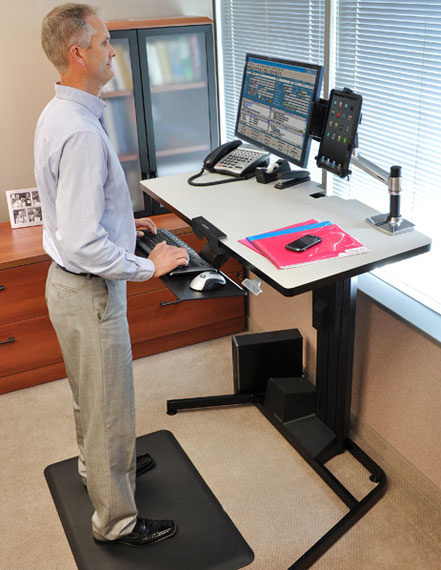购买了稳定的坐站升降桌就可以享受健康的站式办公了吗？并不是，还有一个健康办公的重要的方面是调节显示屏幕与键盘和鼠标之间的距离。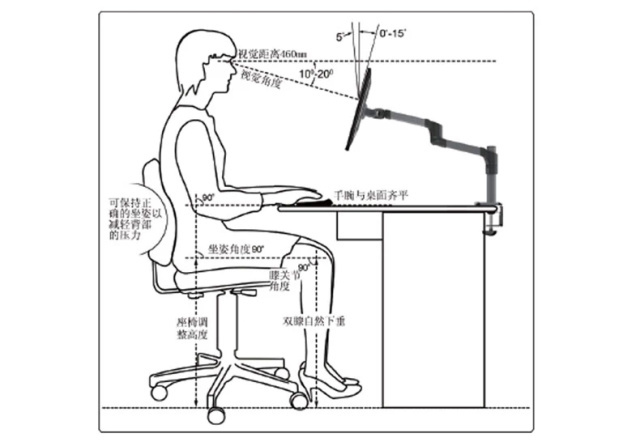显示器距离眼睛的距离约460mm，视觉角度为10－20度。手肘的夹角约为90度直角，手腕与键盘和鼠标所在平面齐平。只有在这个标准姿势下，才能充分打开上身躯干，防止弯腰驼背，令呼吸保持顺畅。要想充分拉开显示器和鼠标键盘之间的距离，一般人们都会在升降桌上再配置一个显示器支架调节显示器的高度和角度。如果是笔记本电脑一定要使用外接键盘和鼠标，方能达到最佳效果。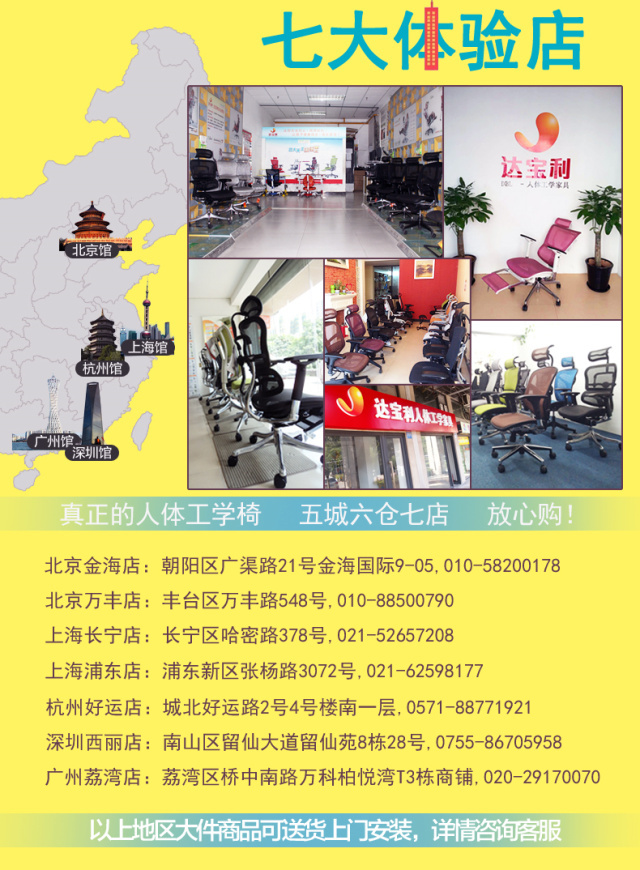`声明：本文由入驻焦点开放平台的作者撰写，除焦点官方账号外，观点仅代表作者本人，不代表焦点立场错误信息举报电话： 400-099-0099，邮箱：jubao@vip.sohu.com，或点此进行意见反馈，或点此进行举报投诉。`A B C D E F G H J K L M N P Q R S T W X Y Z
A - B - C - D - E
• A
• 鞍山
• 安庆
• 安阳
• 安顺
• 安康
• 澳门
• B
• 北京
• 保定
• 包头
• 巴彦淖尔
• 本溪
• 蚌埠
• 亳州
• 滨州
• 北海
• 百色
• 巴中
• 毕节
• 保山
• 宝鸡
• 白银
• 巴州
• C
• 承德
• 沧州
• 长治
• 赤峰
• 朝阳
• 长春
• 常州
• 滁州
• 池州
• 长沙
• 常德
• 郴州
• 潮州
• 崇左
• 重庆
• 成都
• 楚雄
• 昌都
• 慈溪
• 常熟
• D
• 大同
• 大连
• 丹东
• 大庆
• 东营
• 德州
• 东莞
• 德阳
• 达州
• 大理
• 德宏
• 定西
• 儋州
• 东平
• E
• 鄂尔多斯
• 鄂州
• 恩施
F - G - H - I - J
• F
• 抚顺
• 阜新
• 阜阳
• 福州
• 抚州
• 佛山
• 防城港
• G
• 赣州
• 广州
• 桂林
• 贵港
• 广元
• 广安
• 贵阳
• 固原
• H
• 邯郸
• 衡水
• 呼和浩特
• 呼伦贝尔
• 葫芦岛
• 哈尔滨
• 黑河
• 淮安
• 杭州
• 湖州
• 合肥
• 淮南
• 淮北
• 黄山
• 菏泽
• 鹤壁
• 黄石
• 黄冈
• 衡阳
• 怀化
• 惠州
• 河源
• 贺州
• 河池
• 海口
• 红河
• 汉中
• 海东
• I
• J
• 晋中
• 锦州
• 吉林
• 鸡西
• 佳木斯
• 嘉兴
• 金华
• 景德镇
• 九江
• 吉安
• 济南
• 济宁
• 焦作
• 荆门
• 荆州
• 江门
• 揭阳
• 金昌
• 酒泉
• 嘉峪关
K - L - M - N - P
• K
• 开封
• 昆明
• 昆山
• L
• 廊坊
• 临汾
• 辽阳
• 连云港
• 丽水
• 六安
• 龙岩
• 莱芜
• 临沂
• 聊城
• 洛阳
• 漯河
• 娄底
• 柳州
• 来宾
• 泸州
• 乐山
• 六盘水
• 丽江
• 临沧
• 拉萨
• 林芝
• 兰州
• 陇南
• M
• 牡丹江
• 马鞍山
• 茂名
• 梅州
• 绵阳
• 眉山
• N
• 南京
• 南通
• 宁波
• 南平
• 宁德
• 南昌
• 南阳
• 南宁
• 内江
• 南充
• P
• 盘锦
• 莆田
• 平顶山
• 濮阳
• 攀枝花
• 普洱
• 平凉
Q - R - S - T - W
• Q
• 秦皇岛
• 齐齐哈尔
• 衢州
• 泉州
• 青岛
• 清远
• 钦州
• 黔南
• 曲靖
• 庆阳
• R
• 日照
• 日喀则
• S
• 石家庄
• 沈阳
• 双鸭山
• 绥化
• 上海
• 苏州
• 宿迁
• 绍兴
• 宿州
• 三明
• 上饶
• 三门峡
• 商丘
• 十堰
• 随州
• 邵阳
• 韶关
• 深圳
• 汕头
• 汕尾
• 三亚
• 三沙
• 遂宁
• 山南
• 商洛
• 石嘴山
• T
• 天津
• 唐山
• 太原
• 通辽
• 铁岭
• 泰州
• 台州
• 铜陵
• 泰安
• 铜仁
• 铜川
• 天水
• 天门
• W
• 乌海
• 乌兰察布
• 无锡
• 温州
• 芜湖
• 潍坊
• 威海
• 武汉
• 梧州
• 渭南
• 武威
• 吴忠
• 乌鲁木齐
X - Y - Z
• X
• 邢台
• 徐州
• 宣城
• 厦门
• 新乡
• 许昌
• 信阳
• 襄阳
• 孝感
• 咸宁
• 湘潭
• 湘西
• 西双版纳
• 西安
• 咸阳
• 西宁
• 仙桃
• 西昌
• Y
• 运城
• 营口
• 盐城
• 扬州
• 鹰潭
• 宜春
• 烟台
• 宜昌
• 岳阳
• 益阳
• 永州
• 阳江
• 云浮
• 玉林
• 宜宾
• 雅安
• 玉溪
• 延安
• 榆林
• 银川
• Z
• 张家口
• 镇江
• 舟山
• 漳州
• 淄博
• 枣庄
• 郑州
• 周口
• 驻马店
• 株洲
• 张家界
• 珠海
• 湛江
• 肇庆
• 中山
• 自贡
• 资阳
• 遵义
• 昭通
• 张掖
• 中卫

1室1厅1厨1卫1阳台

1
2
3
4
5

0
1
2

1

1

0
1
2
3报名成功，资料已提交审核A B C D E F G H J K L M N P Q R S T W X Y Z
A - B - C - D - E
• A
• 鞍山
• 安庆
• 安阳
• 安顺
• 安康
• 澳门
• B
• 北京
• 保定
• 包头
• 巴彦淖尔
• 本溪
• 蚌埠
• 亳州
• 滨州
• 北海
• 百色
• 巴中
• 毕节
• 保山
• 宝鸡
• 白银
• 巴州
• C
• 承德
• 沧州
• 长治
• 赤峰
• 朝阳
• 长春
• 常州
• 滁州
• 池州
• 长沙
• 常德
• 郴州
• 潮州
• 崇左
• 重庆
• 成都
• 楚雄
• 昌都
• 慈溪
• 常熟
• D
• 大同
• 大连
• 丹东
• 大庆
• 东营
• 德州
• 东莞
• 德阳
• 达州
• 大理
• 德宏
• 定西
• 儋州
• 东平
• E
• 鄂尔多斯
• 鄂州
• 恩施
F - G - H - I - J
• F
• 抚顺
• 阜新
• 阜阳
• 福州
• 抚州
• 佛山
• 防城港
• G
• 赣州
• 广州
• 桂林
• 贵港
• 广元
• 广安
• 贵阳
• 固原
• H
• 邯郸
• 衡水
• 呼和浩特
• 呼伦贝尔
• 葫芦岛
• 哈尔滨
• 黑河
• 淮安
• 杭州
• 湖州
• 合肥
• 淮南
• 淮北
• 黄山
• 菏泽
• 鹤壁
• 黄石
• 黄冈
• 衡阳
• 怀化
• 惠州
• 河源
• 贺州
• 河池
• 海口
• 红河
• 汉中
• 海东
• I
• J
• 晋中
• 锦州
• 吉林
• 鸡西
• 佳木斯
• 嘉兴
• 金华
• 景德镇
• 九江
• 吉安
• 济南
• 济宁
• 焦作
• 荆门
• 荆州
• 江门
• 揭阳
• 金昌
• 酒泉
• 嘉峪关
K - L - M - N - P
• K
• 开封
• 昆明
• 昆山
• L
• 廊坊
• 临汾
• 辽阳
• 连云港
• 丽水
• 六安
• 龙岩
• 莱芜
• 临沂
• 聊城
• 洛阳
• 漯河
• 娄底
• 柳州
• 来宾
• 泸州
• 乐山
• 六盘水
• 丽江
• 临沧
• 拉萨
• 林芝
• 兰州
• 陇南
• M
• 牡丹江
• 马鞍山
• 茂名
• 梅州
• 绵阳
• 眉山
• N
• 南京
• 南通
• 宁波
• 南平
• 宁德
• 南昌
• 南阳
• 南宁
• 内江
• 南充
• P
• 盘锦
• 莆田
• 平顶山
• 濮阳
• 攀枝花
• 普洱
• 平凉
Q - R - S - T - W
• Q
• 秦皇岛
• 齐齐哈尔
• 衢州
• 泉州
• 青岛
• 清远
• 钦州
• 黔南
• 曲靖
• 庆阳
• R
• 日照
• 日喀则
• S
• 石家庄
• 沈阳
• 双鸭山
• 绥化
• 上海
• 苏州
• 宿迁
• 绍兴
• 宿州
• 三明
• 上饶
• 三门峡
• 商丘
• 十堰
• 随州
• 邵阳
• 韶关
• 深圳
• 汕头
• 汕尾
• 三亚
• 三沙
• 遂宁
• 山南
• 商洛
• 石嘴山
• T
• 天津
• 唐山
• 太原
• 通辽
• 铁岭
• 泰州
• 台州
• 铜陵
• 泰安
• 铜仁
• 铜川
• 天水
• 天门
• W
• 乌海
• 乌兰察布
• 无锡
• 温州
• 芜湖
• 潍坊
• 威海
• 武汉
• 梧州
• 渭南
• 武威
• 吴忠
• 乌鲁木齐
X - Y - Z
• X
• 邢台
• 徐州
• 宣城
• 厦门
• 新乡
• 许昌
• 信阳
• 襄阳
• 孝感
• 咸宁
• 湘潭
• 湘西
• 西双版纳
• 西安
• 咸阳
• 西宁
• 仙桃
• 西昌
• Y
• 运城
• 营口
• 盐城
• 扬州
• 鹰潭
• 宜春
• 烟台
• 宜昌
• 岳阳
• 益阳
• 永州
• 阳江
• 云浮
• 玉林
• 宜宾
• 雅安
• 玉溪
• 延安
• 榆林
• 银川
• Z
• 张家口
• 镇江
• 舟山
• 漳州
• 淄博
• 枣庄
• 郑州
• 周口
• 驻马店
• 株洲
• 张家界
• 珠海
• 湛江
• 肇庆
• 中山
• 自贡
• 资阳
• 遵义
• 昭通
• 张掖
• 中卫• 手机• 分享
• 设计
免费设计
• 计算器
装修计算器
• 入驻
合作入驻
• 联系
联系我们
• 置顶
返回顶部